# Crystallographic group

Jump to: navigation, search

A discrete group of motions of an-dimensional Euclidean space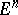having a bounded fundamental domain. Two crystallographic groups are said to be equivalent if they are conjugate in the group of affine transformations of.

The origin of the theory of crystallographic groups is connected with the study of the symmetry of ornaments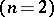and the structure of crystals. A classification of all planar (two-dimensional) and spatial (three-dimensional) crystallographic groups was achieved at the end of the 19th century by E.S. Fedorov and somewhat later by A. Schoenflies (see , , and also , , ). Up to equivalence, there are 17 planar and 219 spatial crystallographic groups; if, however, the spatial groups are considered up to conjugacy with respect to orientation-preserving affine transformations, their number is 230. In 1910, L. Bieberbach investigated crystallographic groups of arbitrary dimension . In particular, he proved the following theorems:

1) Any-dimensional crystallographic groupcontainslinearly independent parallel translations; the groupof linear parts of the transformations fromis finite. (For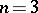this was proved in .)

2) Two crystallographic groups are equivalent if and only if they are isomorphic as abstract groups.

3) For any, there are — up to equivalence — only finitely many-dimensional crystallographic groups (this is a solution to Hilbert's 18th problem).

Theorem 1 yields the following description of the structure of crystallographic groups as abstract groups. Letbe the set of all parallel translations in a crystallographic group. Thenis a normal subgroup of finite index, isomorphic to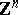, and is its own centralizer in. The existence of a normal subgroupof an abstract grouppossessing these properties is a sufficient condition forto be isomorphic to a crystallographic group .

The groupof linear parts of a crystallographic grouppreserves the lattice; in other words, relative to a basis ofthe transformations inare represented by matrices with integer entries.

In order to specify a crystallographic groupit is necessary to specify — in addition toand— a vector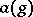for eachsuch that the transformationbelongs to. The vectoris defined up to addition by a vector from. The mappingis a one-dimensional cocycle onwith values in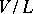, whereis the vector space associated with.

Any triple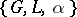, where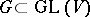is a finite linear group,is a-invariant lattice anda one-dimensional cocycle onwith values in, corresponds as just described with some crystallographic group. Under this correspondence, two triples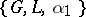and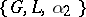, where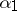and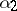are cohomologous cocycles, correspond to equivalent crystallographic groups. To the zero cohomology class corresponds the split (or symmorphic) crystallographic group, which, relative to a suitable choice of an origin, is the set of all transformations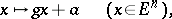where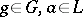.

In matrix interpretation, the description of all-dimensional crystallographic groups reduces to the description of all finite groups of square matrices of orderwith integer entries (up to conjugacy in the group) and, for each such group, to the computation of the cohomology group.

Two cohomology classes define equivalent crystallographic groups if and only if they are transformed into one another by the normalizer ofin. Bieberbach's theorem 2 and a result due to H. Zassenhaus  imply that the natural homomorphism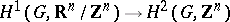is an isomorphism. This is readily deduced from the exact cohomology sequence of.

Two crystallographic groups belong to the same class (arithmetical class) if their groups of linear parts are conjugate in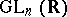(in). Forthere are 32 classes and 73 arithmetical classes of crystallographic groups.

Among the finite groups of matrices with integer entries one can single out the lattice symmetry groups, i.e. the groups of all orthogonal transformations that preserve some fixed lattice in a vector space (and are presented relative to a basis of this lattice). In 1848, A. Bravais determined all possible-dimensional lattice symmetry groups and accordingly divided all-dimensional lattices into 14 types (known as Bravais types). The subgroups ofthat are lattice symmetry groups are called Bravais subgroups.

The Bravais subgroups may also be interpreted as stabilizer subgroups for the natural action ofon the set of positive-definite quadratic forms invariables. They may therefore be determined using reduction theory (see  and Quadratic forms, reduction of). Every maximal finite subgroup of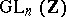is a Bravais subgroup (but the converse is not true).

The following table lists the number of finite subgroups in(up to conjugacy).
<tbody> </tbody>Number of maximal finite subgroups Number of Bravais subgroups Number of finite subgroups 1 1 1 2 2 2 5 13 3 4 14 73 4 9 64 710 5 17 ? ?

An intersection of Bravais subgroups is again a Bravais subgroup. The smallest Bravais subgroupcontaining the groupof linear parts of a crystallographic group, up to conjugacy in(in), is known as the geometrical (arithmetical) holohedry of. Ifis a crystallographic group in general position, in the sense that there is no affine transformation mapping it onto a crystallographic group whose lattice of parallel translations has lower symmetry, then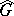is the lattice symmetry group of parallel translations of. Two crystallographic groups belong to the same syngony (Bravais type) if their geometrical (arithmetical) holohedries coincide. Forthere are 7 syngonies and 14 Bravais types of crystallographic groups.

## Linear representations of crystallographic groups.

The irreducible finite-dimensional complex linear representations of a crystallographic groupare described as follows. Letbe some character (a homomorphism into the multiplicative group of complex numbers) of the group, put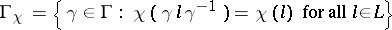and letbe an irreducible representation of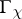such that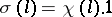, where. Then the representation ofinduced by the representationof its subgroup(see Induced representation) is irreducible. All irreducible representations ofare obtained in this way (see , ).